# Patterns in Context Part D: Counting Stairs (60 minutes)

## Session 2, Part D

Problem D1

Here is a problem in which you can use patterns to make predictions.

Count the number of blocks in each of the following staircases. You can use cubes or blocks to construct the stairs. Then devise as many general methods as you can for predicting the number of blocks in any staircase. If you come up with a rule for predicting the number of blocks, explain why the rule works. If you used a variable in your rule, explain the meaning of the variable.
Note 10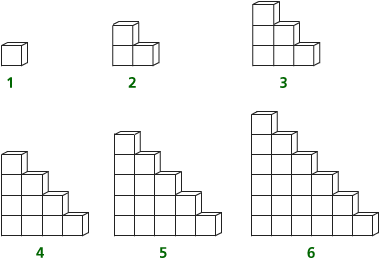Problem D2

If someone tells you how many blocks there are in staircase n, describe how you could use that to find the number of blocks in staircase n + 1.
Note 11

Tip:What is the difference between staircase n and staircase n + 1?

Problem D3

Suppose there are 37,401 blocks in the 273rd staircase. How many blocks are there in the 275th?

Tip: Use what you learned in Problem D2.

Problem D4

How many blocks are there in the 100th staircase?

Tip: As in Part A, try to do this problem by using prediction rather than just by extending the table.

Problem D5

Look at the following geometric solution for the third staircase. Imagine the rectangle made for the nth staircase. Write a rule to determine the number of blocks in the nth staircase.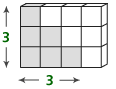### Notes

Note 10

Think about the different conceptions of algebra and the use of variables when working on these problems.

For those not using the Counting Stairs interactive activity, each individual working alone or group should have about 30 cubes to use.

Whether doing the Counting Stairs interactive activity or using blocks or cubes, come up with as many ways as possible for solving the problem. (There are at least 12 different solutions.) If you get stuck, think about building squares or rectangles from the stairs. In fact, the geometric solution to this problem is the most elegant: If you take any staircase with bottom row length n, form a second identical one, rotate it, and put it together with the first, you form a rectangle of dimensions n by n + 1. Therefore, the area of the original staircase is 1/2(n)(n+1).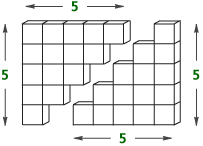Groups: Share answers to Problem D1. If anyone used variables, they should describe what conception of variable they were using.

Note 11

Groups: It is important to talk about Problem D2 as a whole group, as this question underscores the importance and convenience of the recursive formula. If you know the number of stairs in the nth staircase, the number of stairs in the next staircase can be found by adding n + 1 onto the previous total.

Notice that the recursive formula can be applied only in situations where you know the previous term. Part of our job is to determine in which situations different kinds of representations are most useful. What makes a rule useful is how easy it is to apply — closed-form rules often win the battle here — and how easy it is to come up with. In the staircase problem, the recursive rule is much easier to come up with than the closed form.

Another example where the recursive rule is easier to find than the closed-form rule is the famous Fibonacci number sequence: 1, 1, 2, 3, 5, 8, 13, … .

The recursive rule for the sequence is elegant: Start with 1, 1. Then, to get any term in the sequence, add the previous two terms.

The closed-form rule is complicated: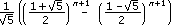It’s not clear that this function produces integer outputs, much less that the outputs are the terms of the sequence above. Imagine trying to come up with such a formula!

### Solutions

Problem D1

One method is that the number of blocks for the nth staircase is n more than the number of blocks for the (n – 1)st staircase, because you need to add on a row of n blocks to build the new staircase. This allows you to construct a table. One clever trick is to piece together 2 consecutive staircases; the 5th staircase fits nicely into the 6th staircase to form a 6-by-6 square!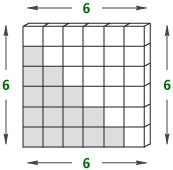Problem D2

There will be 1 new row in staircase n + 1, a row with n + 1 blocks in it. That means that to go from staircase n to staircase n + 1, you will need to add n + 1 blocks.

Problem D3

In the 274th staircase there will be 37,401 + 274 = 37,675 blocks. In the 275th staircase there will be 37,675 + 275 = 37,950 blocks. This answer can also be reached by using (275) * (276) / 2 = 37,950.

Problem D4

There are 5,050 blocks in the 100th staircase. The fastest way is to use n(n + 1) / 2.

Problem D5

A way to build a general formula is to notice that 2 copies of the 6th staircase will form a 6-by-7 rectangle. For the nth staircase this will be n(n + 1) blocks for 2 staircases, which means n(n + 1) / 2 for 1.